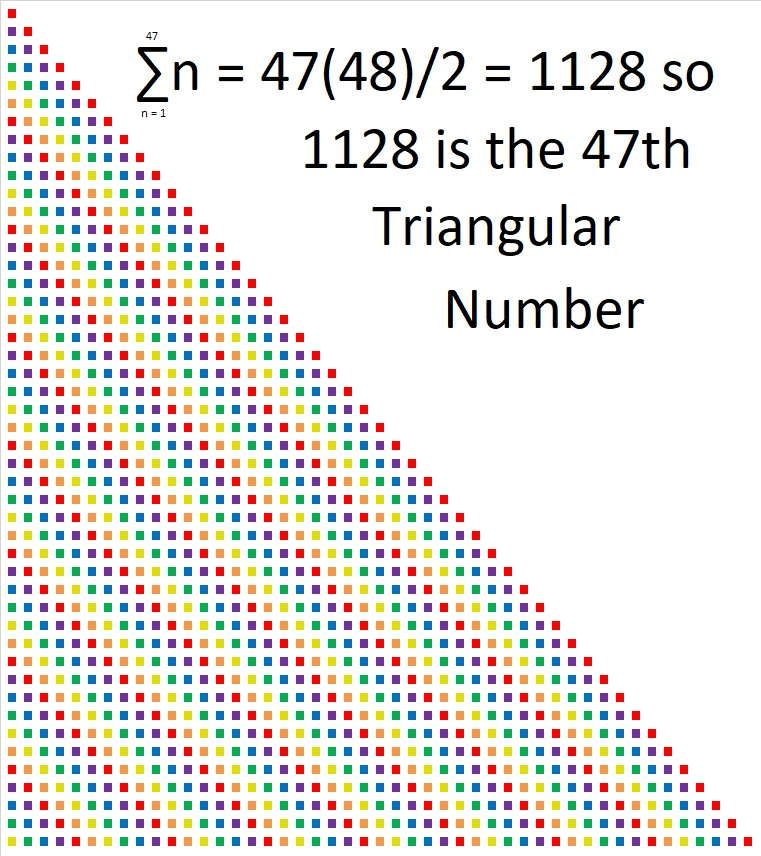# 1128 is the 24th Hexagonal Number

1128 is the 24th hexagonal number because of the way that it can be factored:
2(24²) – 24 = 1128,
(2(24) – 1)24 = 1128
or simply 47(24) = 1128.

This is what the 24th hexagonal number looks like when it is made with 1128 tiny squares arranged into a hexagon:All hexagonal numbers are also triangular numbers. 1128 is the 47th triangular number:What else can the factors of 1128 tell us?

• 1128 is a composite number.
• Prime factorization: 1128 = 2 × 2 × 2 × 3 × 47, which can be written 1128 = 2³ × 3 × 47
• The exponents in the prime factorization are 3, 1, and 1. Adding one to each and multiplying we get (3 + 1)(1 + 1)(1 + 1) = 4 × 2 × 2 = 16. Therefore 1128 has exactly 16 factors.
• Factors of 1128: 1, 2, 3, 4, 6, 8, 12, 24, 47, 94, 141, 188, 282, 376, 564, 1128
• Factor pairs: 1128 = 1 × 1128, 2 × 564, 3 × 376, 4 × 282, 6 × 188, 8 × 141, 12 × 94, or 24 × 47
• Taking the factor pair with the largest square number factor, we get √1128 = (√4)(√282) = 2√282 ≈ 33.58571This site uses Akismet to reduce spam. Learn how your comment data is processed.